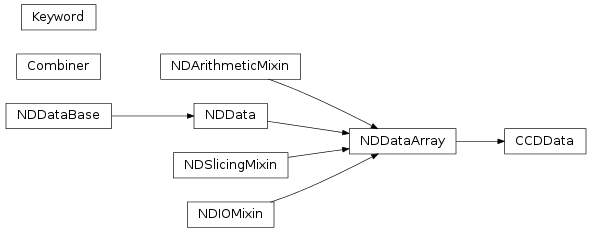# CCD Data reduction (ccdproc)¶

## Introduction¶

Note

ccdproc works only with astropy version 0.4.0 or later.

The ccdproc package provides:

• An image class, CCDData, that includes an uncertainty for the data, units and methods for performing arithmetic with images including the propagation of uncertainties.
• A set of functions performing common CCD data reduction steps (e.g. dark subtraction, flat field correction) with a flexible mechanism for logging reduction steps in the image metadata.
• A class for combining and/or clipping images, Combiner, and associated functions.

## Getting Started¶

Warning

ccdproc is still under active development. The API will almost certainly change.

In addition, testing of ccdproc on real data is currently very limited. Use with caution, and please report any errors you find at the GitHub repo for this project.

A CCDData object can be created from a numpy array (masked or not) or from a FITS file:

```>>> import numpy as np
>>> from astropy import units as u
>>> import ccdproc
>>> image_1 = ccdproc.CCDData(np.ones((10, 10)), unit="adu")
```

An example of reading from a FITS file is image_2 = ccdproc.CCDData.read('my_image.fits', unit="electron") (the electron unit is defined as part of ccdproc).

The metadata of a CCDData object may be any dictionary-like object, including a FITS header. When a CCDData object is initialized from FITS file its metadata is a FITS header.

The data is accessible either by indexing directly or through the data attribute:

```>>> sub_image = image_1[:, 1:-3]  # a CCDData object
>>> sub_data =  image_1.data[:, 1:-3]  # a numpy array
```

See the documentation for CCDData for a complete list of attributes.

Most operations are performed by functions in ccdproc:

```>>> dark = ccdproc.CCDData(np.random.normal(size=(10, 10)), unit="adu")
>>> dark_sub = ccdproc.subtract_dark(image_1, dark,
...                                  dark_exposure=30*u.second,
...                                  data_exposure=15*u.second,
...                                  scale=True)
```

Every function returns a copy of the data with the operation performed. If, for some reason, you wanted to modify the data in-place, do this:

```>>> image_2 = ccdproc.subtract_dark(image_1, dark, dark_exposure=30*u.second, data_exposure=15*u.second, scale=True)
```

See the documentation for subtract_dark for more compact ways of providing exposure times.

Every function in ccdproc supports logging through the addition of information to the image metadata.

```>>> image_2_gained = ccdproc.gain_correct(image_2, 1.5 * u.photon/u.adu, add_keyword='gain_corrected')
```

Logging can be more complicated – add several keyword/value pairs by passing a dictionary to add_keyword:

```>>> my_log = {'gain_correct': 'Gain value was 1.5',
...           'calstat': 'G'}
>>> image_2_gained = ccdproc.gain_correct(image_2,
```

The Keyword class provides a compromise between the simple and complicated cases for providing a single key/value pair:

```>>> key = ccdproc.Keyword('gain_corrected', value='Yes')
>>> image_2_gained = ccdproc.gain_correct(image_2,
```

Keyword also provides a convenient way to get a value from image metadata and specify its unit:

```>>> image_2.header['gain']  = 1.5
>>> image_2_var = ccdproc.create_deviation(image_2,
```

You might wonder why there is a gain_correct at all, since the implemented gain correction simple multiplies by a constant. There are two things you get with gain_correct that you do not get with multiplication:

• Appropriate scaling of uncertainties.
• Units

The same advantages apply to operations that are more complex, like flat correction, in which one image is divided by another:

```>>> flat = ccdproc.CCDData(np.random.normal(1.0, scale=0.1, size=(10, 10)),
>>> image_1_flat = ccdproc.flat_correct(image_1, flat)
```

In addition to doing the necessary division, flat_correct propagates uncertainties (if they are set).

## ccdproc Module¶

The ccdproc package is a collection of code that will be helpful in basic CCD processing. These steps will allow reduction of basic CCD data as either a stand-alone processing or as part of a pipeline.

### Functions¶

 background_deviation_box(data, bbox) Determine the background deviation with a box size of bbox. background_deviation_filter(data, bbox) Determine the background deviation for each pixel from a box with size of bbox. cosmicray_lacosmic(ccd[, error_image, ...]) Identify cosmic rays through the lacosmic technique. cosmicray_median(ccd[, error_image, thresh, ...]) Identify cosmic rays through median technique. create_deviation(ccd_data[, gain, ...]) Create a uncertainty frame. flat_correct(ccd, flat[, min_value, add_keyword]) Correct the image for flat fielding. gain_correct(ccd, gain[, gain_unit, add_keyword]) Correct the gain in the image. rebin(ccd, newshape) Rebin an array to have a new shape. sigma_func(arr) Robust method for calculating the deviation of an array. subtract_bias(ccd, master[, add_keyword]) Subtract master bias from image. subtract_dark(ccd, master[, dark_exposure, ...]) Subtract dark current from an image. subtract_overscan(ccd[, overscan, ...]) Subtract the overscan region from an image. test([package, test_path, args, plugins, ...]) Run the tests using py.test. transform_image(ccd, transform_func[, ...]) Transform the image Using the function specified by transform_func, the transform will be applied to data, uncertainty, and mask in ccd. trim_image(ccd[, fits_section, add_keyword]) Trim the image to the dimensions indicated.

### Classes¶

 CCDData(*args, **kwd) A class describing basic CCD data The CCDData class is based on the NDData object and includes a data array, uncertainty frame, mask frame, meta data, units, and WCS information for a single CCD image. Combiner(ccd_list[, dtype]) A class for combining CCDData objects. Keyword(name[, unit, value])

### Class Inheritance Diagram¶